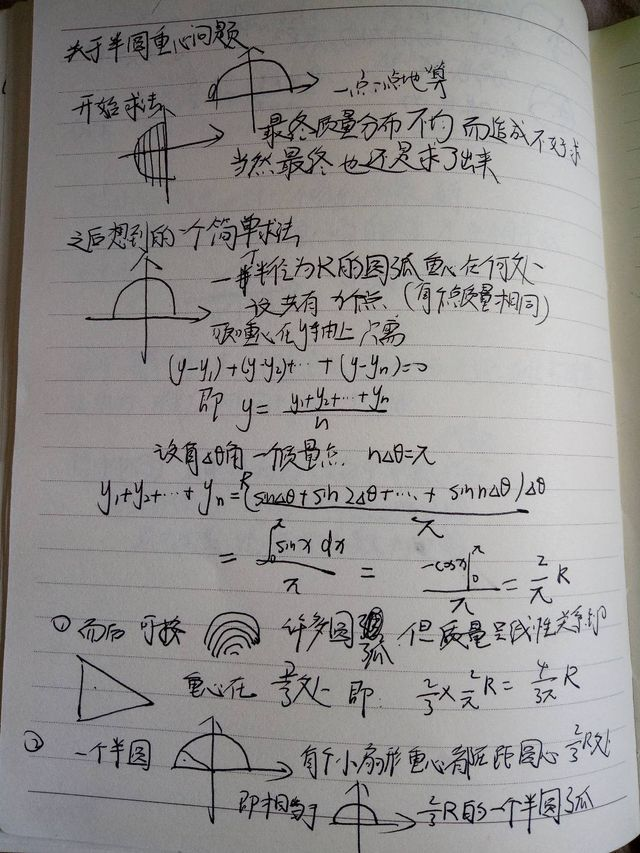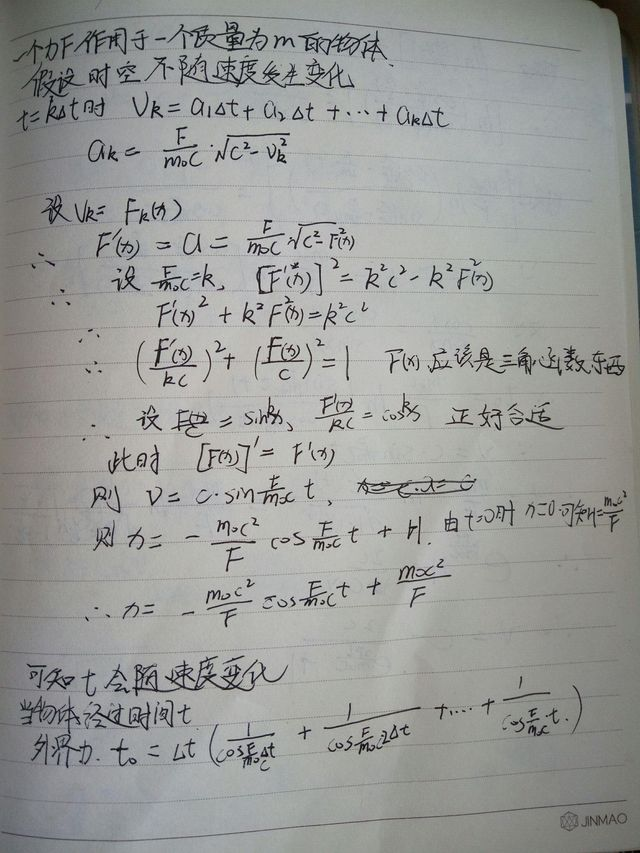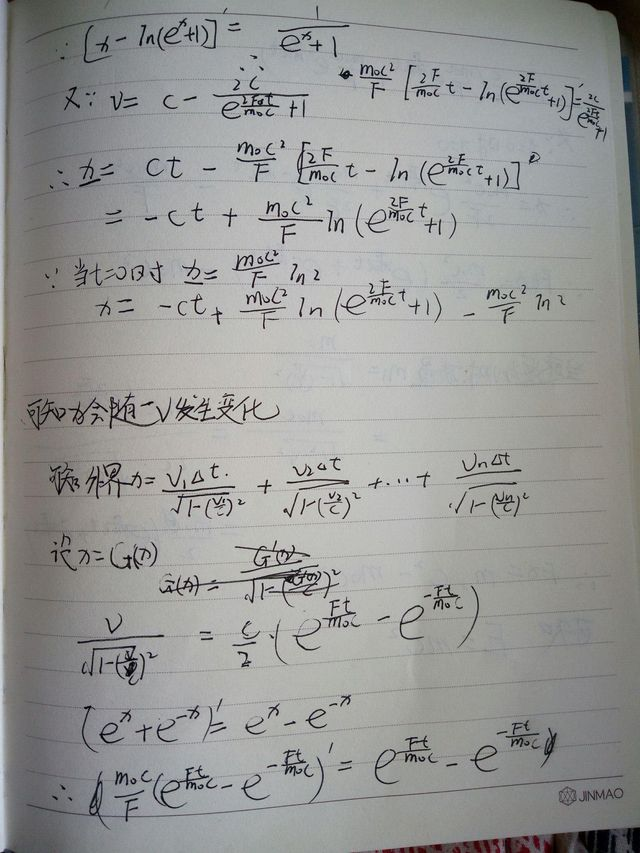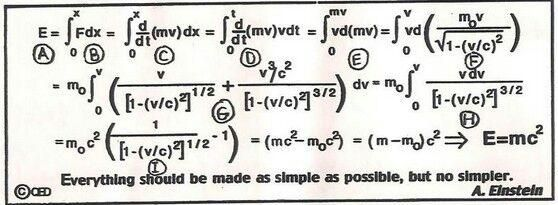## 关于我在高中想成为物理学家这件事

2023-07-04

ps. 请原谅我那被老师评价为鸡爪子挠一样的字

### 小球下落速度

$V_n= \frac{1 - e^{-\frac{k}{m}t}}{\frac{k}{mg}}$### 半圆重心在哪里### $E=mc^2$

$T={\frac {T_{0}}{{\sqrt {1-({\frac {v}{c}})^{2}}}}}$
$L=L_{0}{\sqrt {1-\left({\frac {v}{c}}\right)^{2}}}$
$m={\frac {m_{0}}{{\sqrt {1-\left({\frac {v}{c}}\right)^{2}}}}}$
$E=mc^2$## 关于这些

> 高中三年算出来一些挺有意思的问题，并且高中物理蛮好(尽管高考物理并不出色 T.T)

## 时空穿梭

> 是啊，你安安稳稳活到了现在，有着自己的收入来源。

> 写代码这件事你很喜欢，特别是在写自己项目的时候。

> 你所担心的神经衰弱，所带给你的每一次惊吓，竟然很久都没再出现过。

> 认识的人，他们总是热情又认真。---The End---